# Motion Graphs Interpret The Graph Below Interpret The

• Slides: 29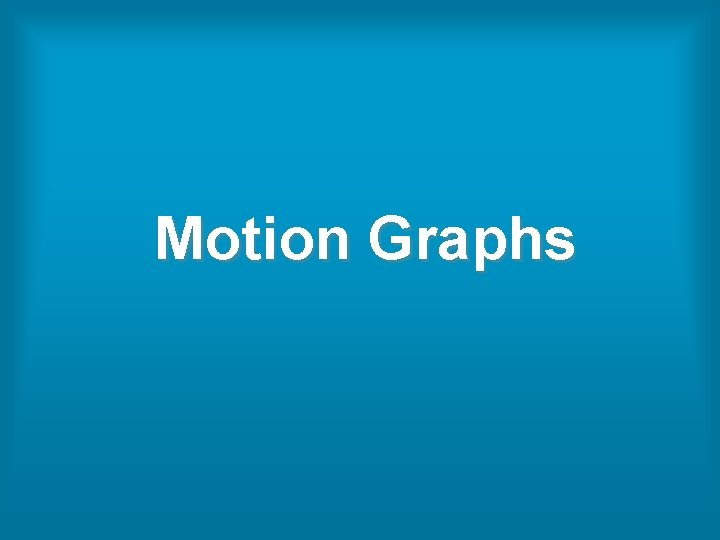Motion Graphs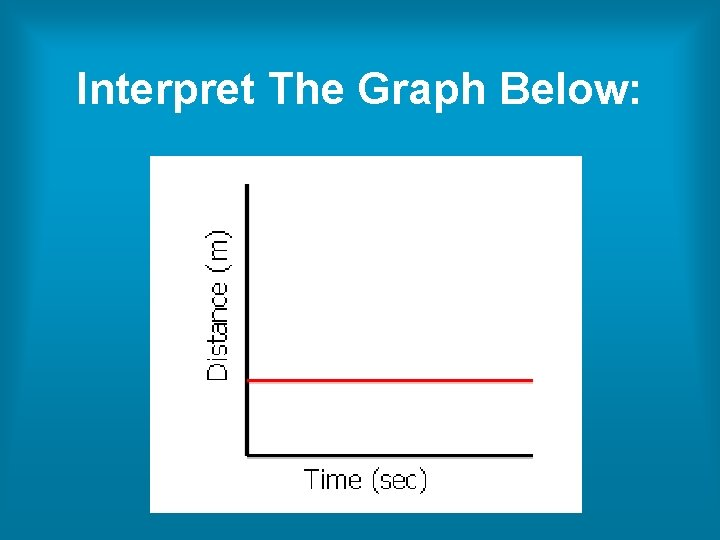Interpret The Graph Below: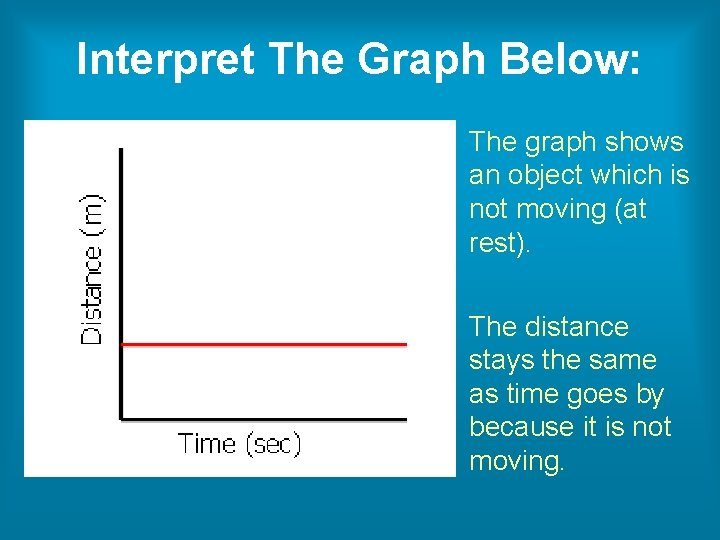Interpret The Graph Below: The graph shows an object which is not moving (at rest). The distance stays the same as time goes by because it is not moving.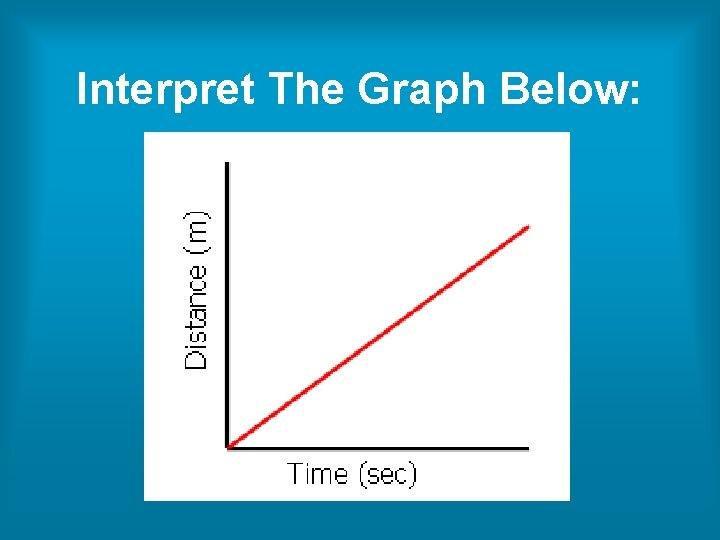Interpret The Graph Below: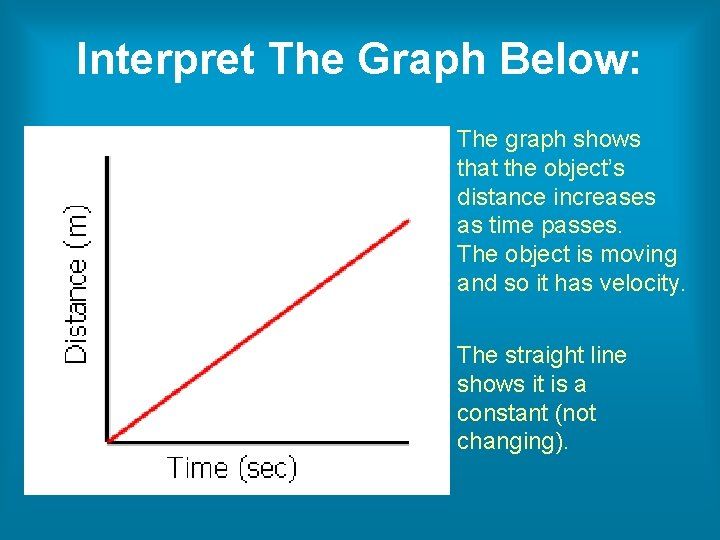Interpret The Graph Below: The graph shows that the object’s distance increases as time passes. The object is moving and so it has velocity. The straight line shows it is a constant (not changing).Interpret The Graph Below:Interpret The Graph Below: The curve in the graph shows that the objects velocity is changing as time passes. This is acceleration.Interpret The Graph Below: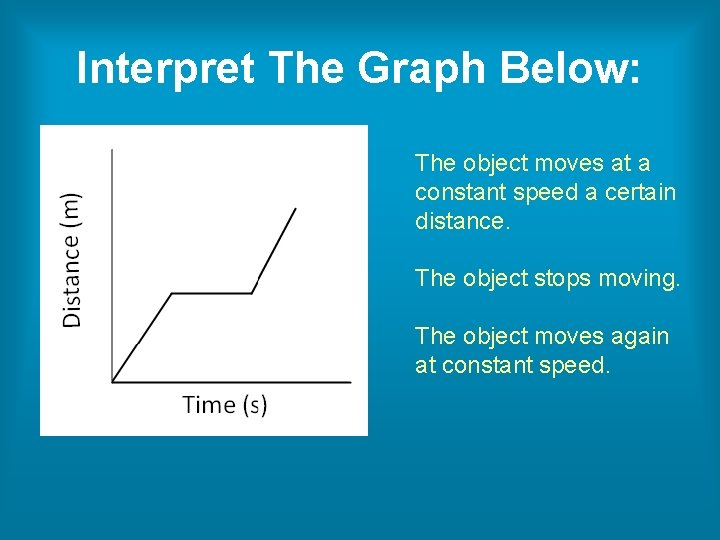Interpret The Graph Below: The object moves at a constant speed a certain distance. The object stops moving. The object moves again at constant speed.Interpret The Graph Below: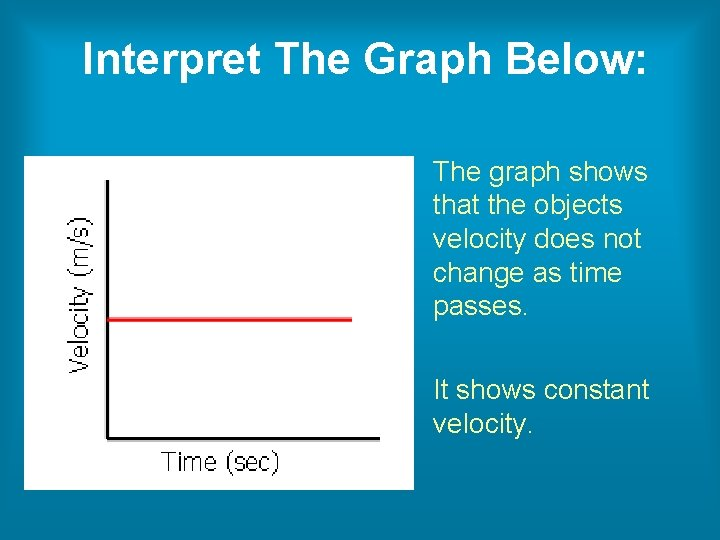Interpret The Graph Below: The graph shows that the objects velocity does not change as time passes. It shows constant velocity.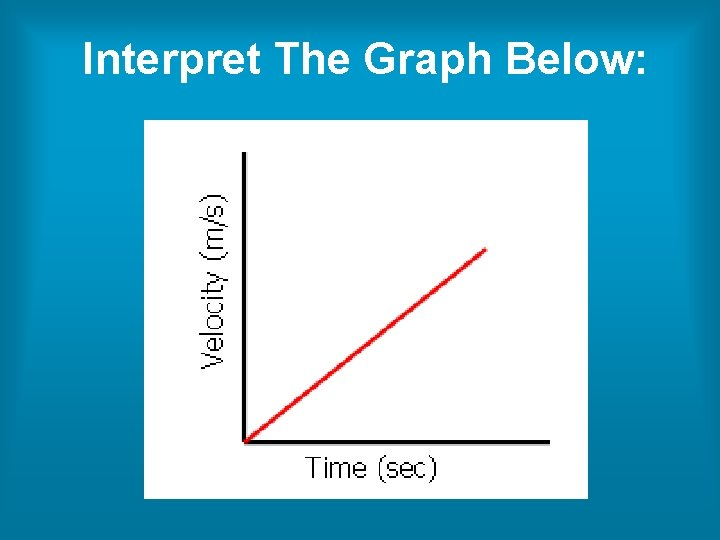Interpret The Graph Below: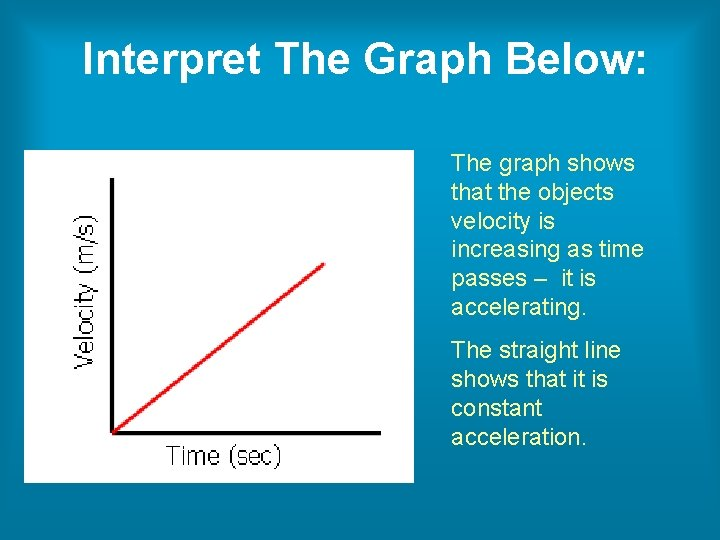Interpret The Graph Below: The graph shows that the objects velocity is increasing as time passes – it is accelerating. The straight line shows that it is constant acceleration.Interpret The Graph Below: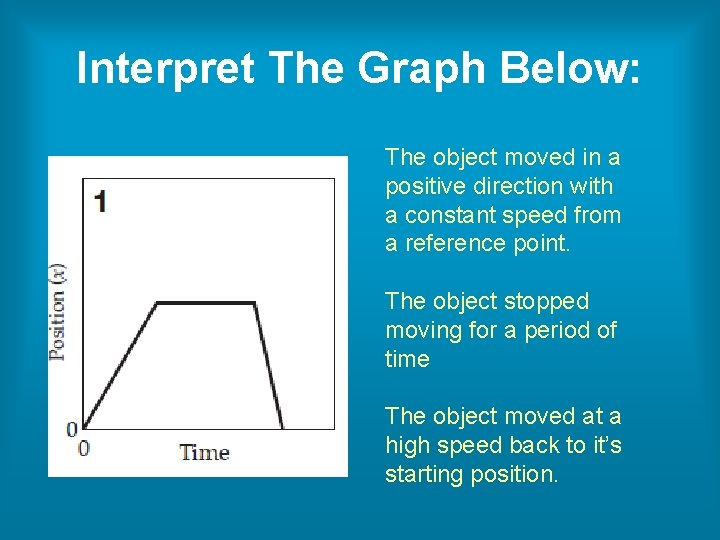Interpret The Graph Below: The object moved in a positive direction with a constant speed from a reference point. The object stopped moving for a period of time The object moved at a high speed back to it’s starting position.Interpret The Graph Below:Interpret The Graph Below: The object moved quickly in a positive direction from the reference point. The object stopped for a period of time. The object continued even faster in the positive direction, away from the reference point.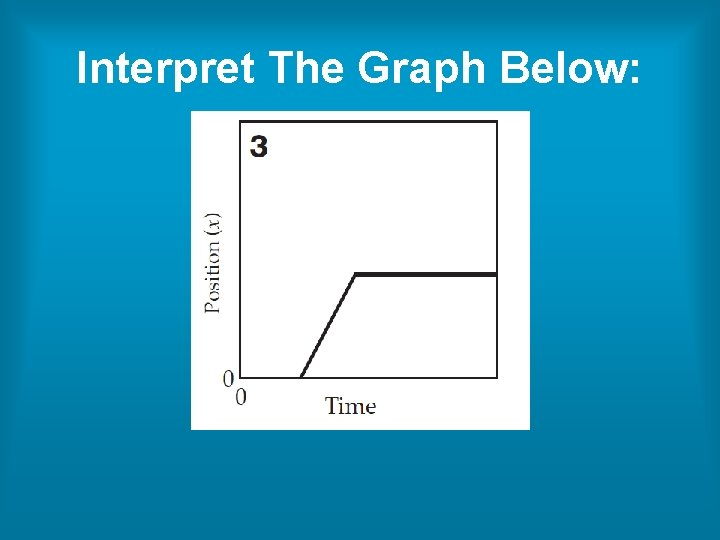Interpret The Graph Below:Interpret The Graph Below: The object starts from the reference point some time after the clock starts. The object stops and doesn’t move again.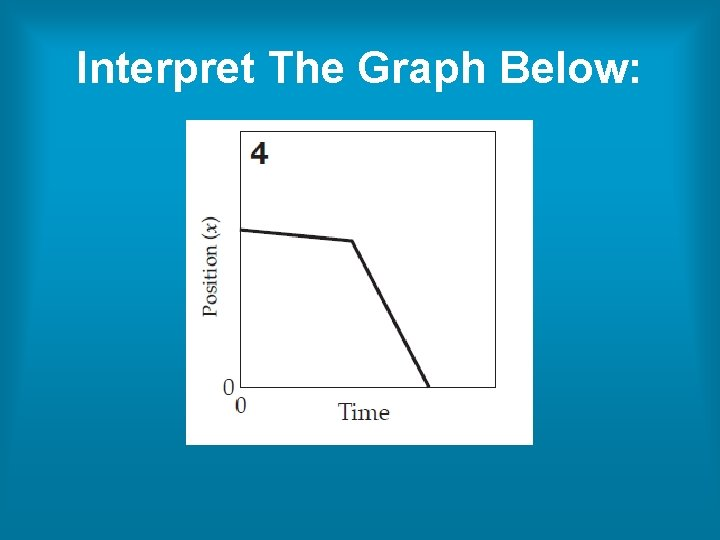Interpret The Graph Below:Interpret The Graph Below: The object starts at a position some distance away from the reference point. The object moves slowly in the negative direction (towards reference point). The object moves quickly toward the reference point in the negative direction.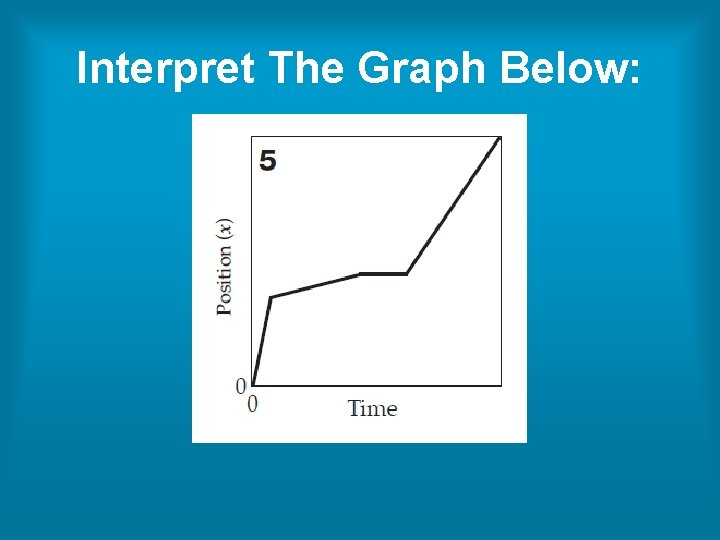Interpret The Graph Below: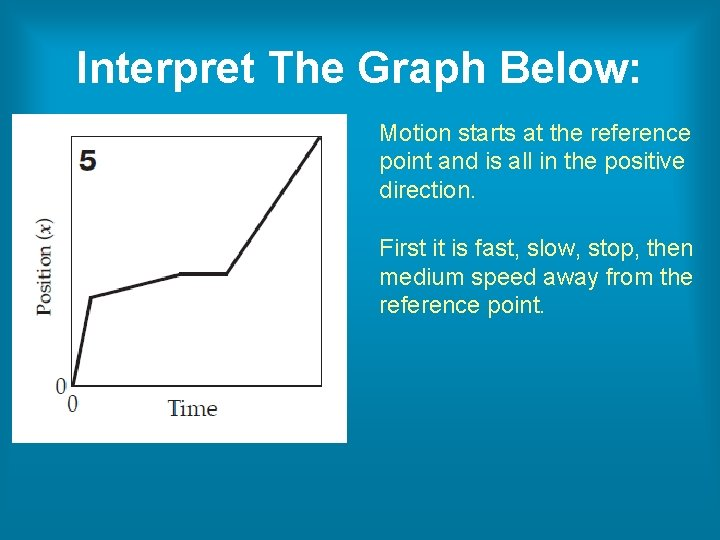Interpret The Graph Below: Motion starts at the reference point and is all in the positive direction. First it is fast, slow, stop, then medium speed away from the reference point.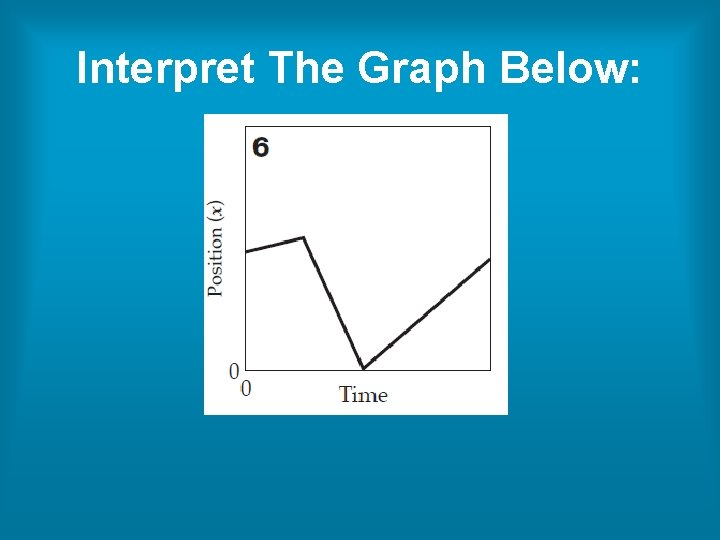Interpret The Graph Below:Interpret The Graph Below: Motion starts in the positive direction, slowly. Motion is in high speed in the negative direction until the object is back at the reference point. Then medium speed in the positive direction.Interpret The Graph Below:Interpret The Graph Below: Just like the previous graph, this graph shows an object moving with constant velocityInterpret The Graph Below:Interpret The Graph Below: In the first part of the graph the object is moving with constant velocity. In the second part of the graph the object is at rest (not moving). In the third part the object is again moving with constant velocity.